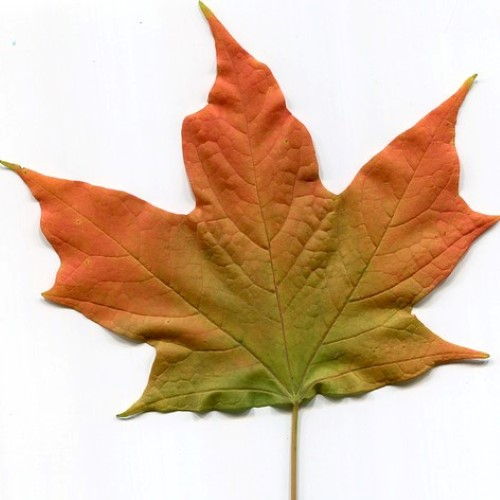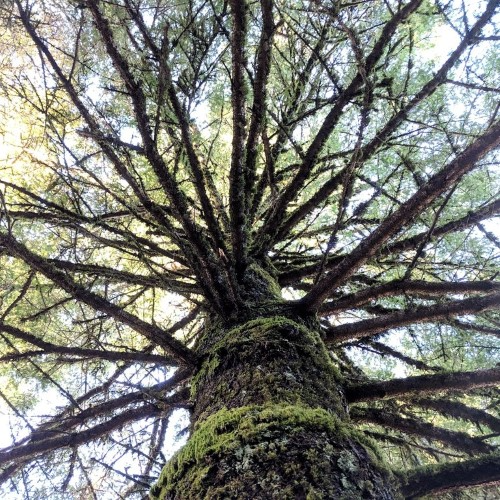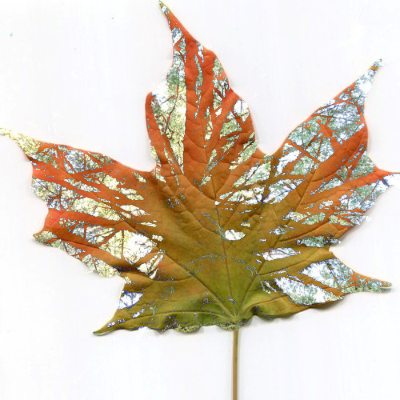# Image Blender

example p5.js javascript images 🎃

This sketch takes two images and blends them together by taking the average of each pixel.The sketch blends them together to create this image:``````let imgOne;
let imgTwo;

}

function setup() {
createCanvas(500, 500);

// Resize the images so they fit on the screen
imgOne.resize(width, height);
imgTwo.resize(width, height);

// other setup
noLoop();
noSmooth();
}

function draw() {

// Look at each pixel in the images,
// and average their R, G, and B values
// to mix the colors together.
for (let y = 0; y < imgOne.height; y++) {
for (let x = 0; x < imgOne.width; x++) {

// Get the colors.
const colorOne = imgOne.get(x, y);
const colorTwo = imgTwo.get(x, y);

// Compute average R, G, and B values.
const avgRed = (red(colorOne) + red(colorTwo)) / 2;
const avgGreen = (green(colorOne) + green(colorTwo)) / 2;
const avgBlue = (blue(colorOne) + blue(colorTwo)) / 2;

// Draw a point with the average color.
stroke(avgRed, avgGreen, avgBlue);
point(x, y);
}
}

}

//noprotect
``````

Try changing the code inside the nested `for` loop to blend the images together differently. For example:

``````for (let y = 0; y < imgOne.height; y++) {
for (let x = 0; x < imgOne.width; x++) {

// Get the colors.
const colorOne = imgOne.get(x, y);
const colorTwo = imgTwo.get(x, y);

// Get the brightness.
const brightnessOne = red(colorOne) + green(colorOne) + blue(colorOne);
const brightnessTwo = red(colorTwo) + green(colorTwo) + blue(colorTwo);

// Draw using the brightest color.
if (brightnessOne > brightnessTwo) {
stroke(colorOne);
} else {
stroke(colorTwo);
}
point(x, y);
}
}
``````

This code compares the brightness of each pixel, and draws whichever pixel is brighter. This creates images like this:This is part of p5 spooky sketches printout I made for CC Fest in 2019. That printout contains a bunch of Halloween-themed examples of drawing and image manipulation. Feel free to use it on your own or in a classroom!

# Remix Ideas

• Instead of averaging the pixels, create a color by taking the green from the first image and the red from the second image.
• Take the darker pixel instead of the brighter pixel.
• Try blending together more than two images!